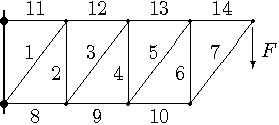# Solving a Truss: Methodial of Joints

## Homework StatementThe truss is suspended by two pin joints. Each segment is 3 m wide and 4 m high. The applied force is F = 6 N. What is the force in member 10? Include the sign for tension (positive) or compression (negative).

## Homework Equations

T=rf
sum of forces = 0

## The Attempt at a Solution

On the far end joint for the Y direction

0=-6-F_seven * sin(53.13) [53.13 becaue arctan(4/3)
F_seven = -21.9344

joint on right of 10 in x direction

0=F_seven*cos(90-53.13)-F_ten
=> F_ten =-17.5 N

Please help me solve this..

## Answers and Replies

haruspex
Science Advisor
Homework Helper
Gold Member
2020 Award
You can get there by writing out equations for every joint, but there's an easier way here.
Just consider the truss as three components: the rigid triangle of 6, 7, and 14; the member 10; and everything else as a rigid assembly. Draw that and see what equations you can find.
(Hint: considering moments about a certain joint will help.)

PhanthomJay
Science Advisor
Homework Helper
Gold Member

## Homework StatementThe truss is suspended by two pin joints. Each segment is 3 m wide and 4 m high. The applied force is F = 6 N. What is the force in member 10? Include the sign for tension (positive) or compression (negative).

## Homework Equations

T=rf
sum of forces = 0

## The Attempt at a Solution

On the far end joint for the Y direction

0=-6-F_seven * sin(53.13) [53.13 becaue arctan(4/3)
F_seven = -21.9344
Your equation is correct but your Math is way off.
joint on right of 10 in x direction

0=F_seven*cos(90-53.13)-F_ten
=> F_ten =-17.5 N
looks like you cosined when you should have sined.
Do you understand the minus sign? It is easier to use method of sections to find force in member 10, as haruspex pointed out. If using method of joints, you should take advantage of the 3-4-5 right triangle to get member forces and components instead of getting bogged down in the trig.

Please help me solve this..[/QUOTE]

Thank you for the responses. I ended up just asking my teacher because I was confused.